## Table of ContentOpen Access

ARTICLE

# A New Three-Parameter Inverse Weibull Distribution with Medical and Engineering Applications

Refah Alotaibi1, Hassan Okasha2,3, Hoda Rezk4, Mazen Nassar2,5,*

1 Department of Mathematical Sciences, College of Science, Princess Nourah bint Abdulrahman University, Riyadh, 11671, Saudi Arabia
2 Department of Statistics, Faculty of Science, King Abdulaziz University, Jeddah, 21589, Saudi Arabia
3 Department of Mathematics, Faculty of Science, Al-Azhar University, Nasr City, 11884, Cairo, Egypt
4 Department of Statistics, Faculty of Commerce, Al-Azhar University, Nasr City, 11884, Cairo, Egypt
5 Department of Statistics, Faculty of Commerce, Zagazig University, Zagazig, 44519, Egypt

* Corresponding Authors: Mazen Nassar. Email:,(This article belongs to this Special Issue: New Trends in Statistical Computing and Data Science)

Computer Modeling in Engineering & Sciences 2023, 135(2), 1255-1274. https://doi.org/10.32604/cmes.2022.022623

## Abstract

The objective of this article is to provide a novel extension of the conventional inverse Weibull distribution that adds an extra shape parameter to increase its flexibility. This addition is beneficial in a variety of fields, including reliability, economics, engineering, biomedical science, biological research, environmental studies, and finance. For modeling real data, several expanded classes of distributions have been established. The modified alpha power transformed approach is used to implement the new model. The data matches the new inverse Weibull distribution better than the inverse Weibull distribution and several other competing models. It appears to be a distribution designed to support decreasing or unimodal shaped distributions based on its parameters. Precise expressions for quantiles, moments, incomplete moments, moment generating function, characteristic generating function, and entropy expression are among the determined attributes of the new distribution. The point and interval estimates are studied using the maximum likelihood method. Simulation research is conducted to illustrate the correctness of the theoretical results. Three applications to medical and engineering data are utilized to illustrate the model’s flexibility.

## Keywords

1  Introduction

Recently, many statistical distributions have been proposed by statisticians. The necessity to develop new distributions appear either due to practical investigations or theoretical concerns or both. Many applications in domains including dependability analysis, finance and risk modelling, insurance, and biological sciences, among others, have indicated in recent years that data sets that follow standard distributions are more often the exception than the usual. Because modified distributions are necessary, significant progress has been made in the modification of several classic distributions and their efficient utilization to challenges in these fields. Lately, specifically since 1980, the direction of statisticians to develop new distributions is on adding parameter(s) to some existing distributions or integrating classical distributions, see Lee et al. . This addition of parameter(s) has been demonstrated valuable in analysing tail properties and also for enhancing the goodness of fit of the family under consideration. For more details about the methods of adding parameters, one can refer to Mudholkar et al. , Marshall et al.  and Mahdavi et al. .

The inverse-Weibull (IW) distribution has many vital applications in life testing and reliability studies. The IW distribution bears some other names like Fréchet distribution. The IW distribution is viewed as reciprocal to the usual Weibull distribution, see Drapella  and Mudholkar et al. , it is used to describe the degradation of mechanical components in diesel engines, such as the crankshaft and pistons, see Keller et al. . Several academics proposed several modified variations of the IW distribution to increase its flexibility. Khan  looked at the beta IW distribution, for example. The generalized IW distribution was proposed by de Gusmao et al. , the modified IW distribution was proposed by Khan et al. , the Kumaraswamy generalized IW distribution was proposed by Oluyede et al. , and the Kumaraswamy modified IW distribution was proposed by Aryal et al. . In addition, Okasha et al.  suggested the Marshall-Olkin IW distribution, Basheer  proposed the alpha power IW distribution, Dey et al.  discussed the logarithmic transformed IW distribution, and Afify et al.  suggested the logarithmic transformed IW distribution.

Assume that X is a random variable follows IW distribution with δ as the scale parameter and θ as the shape parameter, where δ,θ>0. Then, the probability density function (PDF) of X can be written as follows:

g(x;δ,θ)=δθxθ1eδxθ,(1)

The cumulative distribution function (CDF) of Eq. (1) is

G(x;δ,θ)=eδxθ,x>0.(2)

See Johnson et al.  for further information on the IW distribution. In this study, we offer a new three-parameter IW distribution utilizing the same idea proposed by Alotaibi et al. . The modified alpha power transformed IW (MAPTIW) distribution is the name given to the new distribution. Two shape parameters and one scale parameter are included. It includes some lifetime distributions, such as IW, inverted exponential and inverse Rayleigh distributions. Recently, The alpha power transformation approach was used by Alotaibi et al.  to suggest a new method for adding a new shape parameter to a baseline distribution. Modified alpha power transformation (MAPT) was the name given to the approach they proposed. The CDF and PDF of MAPT method are expressed as

F(x)=αG(x)1(α1)(1+ααG(x)),α>0,α1(3)

and

f(x)=α1+G(x)log(α)g(x)(α1)(1+ααG(x))2,α>0,α1,(4)

where α is a new shape parameter. This article seeks to furnish a new lifetime model with the lowest number of parameters, yet satisfactory to depict more complicated data patterns; one scale and two shape parameters are involved to accommodate for decreasing and upside-down bathtub-shaped failure rates. The MAPTIW distribution is motivated by the following causes: (1) It is a comprehensive distribution that holds some well-known lifetime distributions. (2) It is established in Section 2 that the MAPTIW distribution can be considered as a mixture of the traditional IW distribution. (3) The MAPTIW distribution can deliver decreasing and unimodal hazard rates which makes it outstanding from some other distributions. (4) The MAPTIW can be viewed as a practical model for modelling skewed data which can not be adequately modelled by other distributions. (5) The MAPTIW distribution furnishes more significant flexibility when compared with many well-known distributions in two real data applications. We seek that it will attract wider applications in medicine, engineering and other fields of research.

The remainder of this article is structured as follows. The MAPTIW distribution is introduced and its mixed representation is derived in Section 2. In Section 3, we will look at some of the MAPTIW’s most important characteristics. Section 4 shows how to estimate unknown parameters using maximum likelihood estimation. We present the simulation results in Section 5 to show how well the estimations performed. Three real-life data sets are used to assess the importance of the MAPTIW distribution in Section 6. Section 7 presents the conclusion.

2  MAPTIW Distribution

We may define the CDF of the MAPTIW distribution in the following form by inserting the CDF of the IW distribution obtained by Eq. (2) into the CDF of the MAPT generator given by Eq. (3)

F(x;α,δ,θ)=αeδxθ1(α1)(1+ααeδxθ),x,α,δ>0,θ>0,α1.(5)

The PDF that corresponds to the CDF in Eq. (5) can be expressed as follows:

f(x;α,δ,θ)=δθlog(α)xθ1eδxθα1+eδxθ(α1)(1+ααeδxθ)2,x,α,δ>0,θ>0,θ>0,α1.(6)

MAPTIW distribution has the following special sub-models. If α1, it reduces to the IW distribution. If θ=1 and α1, it reduces to the inverted exponential distribution. If θ=2 and α1, it reduces to the inverse Rayleigh distribution. Also, when θ=1, it reduces to the MAPT-inverted exponential distribution. Finally, when θ=2, it simplifies to the MAPT-Rayleigh distribution. The MAPTIW distribution’s reliability function (RF) and hazard rate function (HRF) are

R(x;α,δ,θ)=1αeδxθ1(α1)(1+ααeδxθ),(7)

and

h(x;α,δ,θ)=θδlog(α)xθ1eδxθαeδxθ(1+ααeδxθ)(ααeδxθ),(8)

respectively. Henceforth, we will use X to refer to the random variable that possesses the PDF in Eq. (6).

The various plots of the PDF of the MAPTIW distribution with δ=1 in all settings and different values for α and θ are shown in Fig. 1. The newly introduced parameter α offered more extra flexibility to the PDF of the MAPTIW distribution, as seen in Fig. 1. The MAPTIW distribution is right-skewed, and because of this, it is more suited to modelling right-skewed data than other competing distributions. The many forms of the HRF of the MAPTIW distribution are shown in Fig. 2. It shows that the MAPTIW distribution’s HRF can be decreasing or unimodal in form.Figure 1: MAPTIW PDF plotsFigure 2: HRF plots for the MAPTIW distribution

The PDF in Eq. (6) might be used to obtain various statistical characteristics of the MAPTIW distribution. Finding the PDF’s mixture structure in Eq. (5) is a simple method. Observe the power series below:

αy=m=0(logα)mm!ym,(9)

where y is a variable and log(α) is a constant, as well as the generalized binomial expansion, which has the form

(1y)a=k=0Γ(j+a)Γ(a)j!yk,|y|<1,k>0,(10)

where y is a variable, a is a constant and Γ(a) is the gamma function defined as Γ(a)=0za1ezdz.

When Eqs. (9) and (10) are applied to the PDF in Eq. (6), the following is a suitable linear representation for the PDF

f(x;α,δ,θ)=m=0ϖmg(x;(m+1)δ,θ),(11)

where g(x;(m+1)δ,θ) is the PDF of the IW distribution with scale parameter (m+1)δ and shape parameter θ, and

ϖm=k=0α(k+1)m+1(logα)m+1(α1)(α+1)k+2(m+1)!.

On the other hand, the expansion of the CDF in Eq. (5) may be provided as by integrating Eq. (11) as

F(x;α,δ,θ)=m=0ϖmG(x;(m+1)δ,θ).(12)

Hence G(x;(m+1)δ,θ) is the CDF corresponding to g(x;(m+1)δ,θ).

3  MAPTIW Distribution’s Properties

The quantile function, moments and stress-strength parameter are some of the properties of the MAPTIW distribution that we derive in this part.

3.1 Quantile Function

The quantile function for the MAPTIW distribution may be calculated as follows using Eq. (5):

xu=QMAPIW(u)=[1δlog{[log(1+u(α21)1+u(α1))logα]}]1θ,0<u<1.(13)

One can employ Eq. (13) to simulate the random variable X by taking uUniform(0,1).

3.2 Moments

Moments play an essential part in statistics. Numerous vital aspects of any statistical distribution can be explored via moments. For the MAPTIW distribution, the nth moment can be obtained as

μn=E(Xn)=xnf(x)dx=m=0ϖm0xng(x;(m+1)δ,θ)dx=m=0ϖm[(m+1)δ]nθΓ(1nθ),nθ1,n=1,2,3,,n,

where Γ(.) is the gamma function. In particular, the first two moments can be obtained as

μ1=E(X)=m=0ϖm[(m+1)δ]1θΓ(11θ),θ1,

and

μ2=E(X2)=m=0ϖm[(m+1)δ]2θΓ(12θ),θ2,

The following formulae can also be used to obtain the variance (var), skewness (Sk), and kurtosis (Ku):

var(X)=μ2(μ1)2,

Sk=[μ33μ2μ+2μ3]/[μ2μ2]3/2,

and

Ku=[μ44μ3μ+6μ2μ23μ4]/[μ2μ2]2.

where μ=μ1. The propositions that follow are an explanation of three different types of moments, namely, incomplete moments (IMs), moment generating function (MGF), characteristic generating function (CGF) and conditional moments (CMs).

Prop 3.1. If XMAPTIW, then the IMs of X is given as follows:

ψr(t)=m=0ϖm[(m+1)δ]rθγ(1rθ,(m+1)δtθ),r<θ,(14)

where γ(.,.) is the incomplete gamma function.

Prop 3.2. If XMAPTIW, then the MGF, denoted by M(t), and the cumulant generating function, denoted by K(t), of X are, respectively, given by

MX(t)=k=0m=0ϖmtkk![(m+1)δ]kθΓ(1kθ),

and

KX(t)=log[k=0m=0ϖmtkk!((m+1)δ)kθΓ(1kθ)].

Prop 3.3. If XMAPTIW, then the CGF of X is

MX(t)=k=0m=0ϖm(it)kk![(m+1)δ]kθΓ(1kθ),k<θ.

Prop 3.4. If XMAPTIW, then the CMs of X is

CX(t)=E(Xn|X>x)=ψn(x)1F(x),

where ψn(t) is the nth IM of X.

3.3 Entropies of MAPTIW Distribution

Entropy is used to measure uncertainty. It recreates a vital role in the area of engineering, probability, statistics, information theory and financial analysis. For example, Gençay et al.  delivered a relative analysis of the stock market during the 1987 and 2008 financial crises. Rashidi et al.  examined and simulated the heat transfer flow employing entropy generation in solar still. The Rényi entropy (RE) for the MAPTIW distribution may be calculated using Eq. (6) as follows:

Iρ(x)=11ρlog{(δθlogαα1)ρ0xρ(θ+1)α(1+eδxθ)ρeδρxθ(1+ααeδxθ)2ρdx}=11ρlog{(δθlogαα1)ρs=0r=0(2ρ+s1s)(ρlogα)rr!(1+α)2ρ+s0xρ(θ+1)eδρxθ}×[1+s+ρρeδxθ]rdx=11ρlog{(δθlogαα1)ρs=0r=0u=0r(ru)(2ρ+s1s)(ρlogα)rr!(1+α)2ρ+s(s+ρρ)u×0xρ(θ+1)e(u+1)δxθdx}=11ρlog{(δθlogαα1)ρs=0r=0u=0rv=0(1)vv!(ru)(2ρ+s1s)(ρlogα)rr!(1+α)2ρ+s(s+ρρ)u×Γ(ρ(θ+1)1θ)[(u+1)δ]ρ(θ+1)1θ}.(15)

The Shannon entropy can also be calculated as

E[logf(X)]=log[δθlogαα1]+(θ+1)E[logx]+δE[xθ](logα){1+E[eδxθ]}+2E[log(1+ααeδxθ)]=log[δθlogαα1](θ+1)θm=0ϖm(log(δ(m+1))+γ)δm=0ϖmm+1(logα){1m=0ϖm(m+1)m+2}+2E[log(1+ααeδxθ)].

3.4 Order Statistics

Let X1,,Xn Â be a MAPTIW random sample of size n. Assume that X(1),,X(n) Â are the corresponding order statistics. The ith order statistic’s PDF is then presented as follows:

fX(i)(x)=f(x)B(i,ni+1)a=0ni(1)a(nia)Fj+i1(x),(16)

The beta function is represented as B(.,.). Using Eqs. (5), (6) and after some simplifications, we can obtain

fX(i)(x)=l=0ωlg(x;(l+1)δ,θ),(17)

where g(x;(l+1)δ,θ) is the PDF of the IW distribution with scale parameter (l+1)δ and shape parameter θ, and

ωl=αlogα(α21)i+jB(i,ns+1)a=0nik,m,n=0(1)i+j+m+n+a2(α+1)k+1(n+1)((k+m+1)logα)l(l+1)!×Γ(i+j+k+1)Γ(i+j+1)(i+j1m)(nia)(ln).(18)

Using Eq. (17), we can derive the properties of X(i) directly from the properties of Z(l+1), where Z(l+1) follows IW distribution with scale parameter (l+1)δ and shape parameter θ. Also, the qth moments of X(i) can be derive as follows:

E(X(i)q)=l=0ωlE(Z(l+1)q).(19)

3.5 Moments of Residual Life and Reversed Failure Rate Function

The mean residual lifetime has been studied by engineers and survival analysts. Given a feature or a system is of age t, the remaining lifetime after t is a random variable. The expected value of this random residual lifetime is named the mean residual life. The mean residual lifetime is often a significant measure for discovering an optimal burn-in time for a feature. The rth moment of the residual life of X is given by

Rr(t)=E((Xt)r|X>t)=11F(t)t(xt)rf(x)dx,r1.

Using Eqs. (5) and (6), and the binomial expansion of (xt)r, we get

Rr(t)=11F(t)k=0r(t)rk(rk)txrf(x)dx=11F(t)k=0rm,j=0ϖm,j(t)rk(rk)txrg(x;(m+1)δ,θ)dx=(α1)α{1+(ααeδtθ)1}k=0rm,j=0ϖm,j(t)rk(rk)txrg(x;(m+1)δ,θ)dx.

The mean residual lifetime can be obtained from the last equation by setting r=1. The rth moment of the reversed residual lifetime can be obtained as follows:

mr(t)=E((tX)r|Xt)=1F(t)0t(tx)rf(x)dx,r1.

Based on Eqs. (5), (6) and using the binomial expansion of (tx)r, we can write

mr(t)=(α1)(1+ααeδxθ)αeδxθ1k=0rm,j=0ϖm,j(t)rk(rk)0txrg(x;(m+1)δ,θ)dx.

3.6 Stress-Strength Model

Let Y1MAPTIW(α1,θ1,δ1) and Y2MAPTIW(α2,θ2,δ2). If Y1 describes the stress and Y2 describes the strength, then the MAPTIW distribution’s stress-strength parameter, indicated by R. It has a wide range of applications in engineering and research. We will concentrate on two different cases.

Case one: α1α2, δ1=δ2=δ and θ1=θ2=θ

R=(Y2>Y1)=0F1(y)dF2(y)dy=δθlog(α2)(α11)(α1+1)(α21)(α2+1)20yθ1eδyθα21+eδyθ(α1eδyθ1)×(1α1eδyθα1+1)1(1α2eδyθα2+1)2dy.

Using the series expansion in Eq. (10), it follows:

R=δθlog(α2)(α11)(α21)a=0b=0Ψ1(a,b)0yθ1eδyθ(α1eδyθ1)α1aeδyθα2(b+1)eδyθdy.

Using the series expansion in Eq. (9), we can obtain

R=δθlog(α2)(α11)(α21)a=0b=0Ψ1(a,b)0yθ1eδyθ(α1eδyθ1)α1aeδyθα2(b+1)eδyθdy=δθlog(α2)(α11)(α21)a,b=0c,d=0Ψ1(a,b)T2(c,d)0yθ1e(c+d+1))δyθdy=δθlog(α2)(α11)(α21)a,b=0c,d=0Ψ1(a,b)Ψ2(c,d)(c+d+1))δ,

where

Ψ1(a,b)=(b+1)α2(α1+1)a+1(α2+1)b+2

and

Ψ2(c,d)=(b+1)d(log(α1))c(log(α2))dc!d!((a+1)cac).

Case two: α1α2, δ1δ2 and θ1θ2

R=P(Y2>Y1)=0F1(y)dF2(y)dy=δ2θ2log(α2)(α11)(α1+1)(α21)(α2+1)20yθ21eδ2yθ2α21+eδ2yθ2(α1eδ1yθ11)×(1α1eδ1yθ1α1+1)1(1α2eδ2yθ2α2+1)2dy.

Using the series expansion in Eq. (10), we have

R=δ2θ2log(α2)(α11)(α21)j=0k=0Ψ1(a,b)0yθ21eδ2yθ2(α1eδ1yθ11)α1aeδ1yθ1α2(b+1)eδ2yθ2dy.

Using the series expansion in Eq. (9), we can obtain

R=δ2θ2log(α2)(α11)(α21)a=0b=0Ψ1(a,b)0yθ21eδ2yθ2(α1eδ1yθ11)α1aeδ1yθ1α2(b+1)eδ2yθ2dy=δ2θ2log(α2)(α11)(α21)a,b=0c,d=0Ψ1(a,b)Ψ2(c,d)0yθ21e(cδ1yθ1+(d+1)δ2yθ2)dy.

4  Parameter Estimation

In this section, we consider the maximum likelihood approach to estimate the unknown parameters of the MAPTIW distribution. Furthermore, the approximate confidence intervals (ACIs) of the unknown parameters are acquired.

4.1 Maximum Likelihood Estimation

Given a random sample of size n taken from the MAPTIW distribution with PDF given by Eq. (6), we can express the log-likelihood function as

(α,δ)=nlog(δθlogαα1)(θ+1)i=1nlog(xi)δi=1nxiθ+(logα)i=1n(1+eδxiθ)2i=1nlog(1+ααeδxiθ).(20)

The maximum likelihood estimates (MLEs) denoted by α^, δ^ and θ^ can be got by solving the following normal equations simultaneously

α=nα1+nαlog(α)+i=1n(eδxiθ+1)α2i=1nΨα=0,(21)

δ=nδi=1nxiθlog(α)i=1nxiθeδxiθ2i=1nΨδ=0,(22)

and

θ=nθi=1nlog(xi)+δi=1nxiθlog(xi)+δlog(α)i=1nxiθlog(xi)eδxiθ2i=1nΨθ=0,(23)

where

Ψα=1eδxiθαeδxiθ1ααeδxiθ+1,

Ψδ=log(α)xiθeδxiθαeδxiθααeδxiθ+1,

and

Ψθ=δlog(α)xiθlog(xi)eδxiθαeδxiθααeδxiθ+1.

To get the MLEs of α, δ and θ, any numerical technique can be used to solve Eqs. (21)(23). Using the asymptotic properties of the MLEs, we can construct the ACIs of α, δ and θ. It is known that (α,δ,θ)N((α^,δ^,θ^),I01(α,δ,θ)), where I01(α,δ,θ) is the asymptotic variance-covariance of the MLEs. Here, we employ the approximate asymptotic variance-covariance matrix of the MLEs denoted by I01(α^,δ^,θ^) which obtained based on the observed Fisher information matrix to compute the ACIs as follows:

I01(α^,δ^,θ^)=[IααIαδIαθIαδIδδIδθIαθIδθIθθ](α,δ,θ)=(α^,δ^,θ^)1=[var^(α^)cov^(α^,δ^)cov^(α^,θ^)cov^(δ^,α^)var^(δ^)cov^(δ^,θ^)cov^(θ^,α^)cov^(θ^,δ^)var^(θ^)].(24)

where Iαα,Iδα=Iαδ,Iαθ=Iθα,Iδθ=Iθδ,Iδδ and Iθθ are derived from Eq. (20) as

Iαα=n(1(α1)2log(α)+1α2log2(α))i=1n(eδxiθ+1)α22i=1nΨαα,

Iδδ=nδ2+log(α)i=1nxi2θeδxiθ2i=1nΨδδ,

Iθθ=nθ2δi=1nxiθlog2(xi)+δlog(α)i=1n(δxiθ)xi2θlog2(xi)eδxiθ2i=1nΨθθ,

Iαδ=1αi=1nxiθeδxiθ2i=1nΨαδ,

Iαθ=δi=1nxiθlog(xi)eδxiθα2i=1nΨαθ,

and

Iδθ=i=1nxiθlog(xi)+log(α)i=1n(xiθδ)xi2θlog(xi)eδxiθ2i=1nΨδθ,

where

ΨΨ(α,δ,θ)=log(ααeδxiθ+1),

Ψααeδxiθ(eδxiθ1)αeδxiθ2ααeδxiθ+1(1eδxiθαeδxiθ1)2(ααeδxiθ+1)2,

Ψθθ=δlog(α)xi2θlog2(xi)e2δxiθαeδxiθ(δ(log(α)+eδxiθ)xiθeδxiθ)α+αeδxiθ1δ2log2(α)xi2θlog2(xi)e2δxiθα2eδxiθ(ααeδxiθ+1)2,

Ψδδ=log(α)xi2θe2δxiθαeδxiθ(log(α)+eδxiθ)α+αeδxiθ1log2(α)xi2θe2δxiθα2eδxiθ(ααeδxiθ+1)2,

Ψαθ=δlog(α)xiθlog(xi)eδxiθαeδxiθ(1eδxiθαeδxiθ1)(ααeδxiθ+1)2+(δxiθlog(xi)e2δxiθαeδxiθ1(log(α)+eδxiθ)ααeδxiθ+1),

Ψαδ=xiθeδxiθαeδxiθ1+log(α)xiθe2δxiθαeδxiθ1ααeδxiθ+1log(α)xiθeδxiθαeδxiθ(1eδxiθαeδxiθ1)(ααeδxiθ+1)2

and

Ψθδ=log(α)xi2θlog(xi)e2δxiθαeδxiθ(δ(log(α)+eδxiθ)xiθeδxiθ)α+αeδxiθ1+δlog2(α)xi2θlog(xi)e2δxiθα2eδxiθ(ααeδxiθ+1)2.

Therefore, the (1γ)%ACIs ofα δ,andθ are as follows:

α^±zγ/2var^(α^),δ^±zγ/2var^(δ^)andθ^±zγ/2var^(θ^),

where zγ/2 is the upper (γ/2)th percentile point of a standard normal distribution.

5  Simulation Study

In this part, we use a simulation with variable sample sizes n and various values of the parameters α,δ,and θ to show how well the MLEs of the MAPTIW distribution perform. The results are based on sample sizes of 20, 50, and 100 with parameter values α=(1.5,3,5), δ=(0.5,1.5,3) and θ=(1.5,3,5), respectively. We get MLEs, mean square errors (MSEs), and confidence lengths (CLs) in each case. The simulation study is implemented using the steps below:

1.    Establish the sample size and parameter initial values.

2.    Create a random sample of size n from the MAPTIW distribution using Eq. (13).

3.    Compute the MLEs of α,δ and θ.

4.    Compute the MSEs of α,δ and θ.

5.    Get the CLs of α,δ and θ.

6.    Redo Steps 2–5, 1000 times.

7.    Determine the average values of estimates (AEs), MSEs, and CLs as follows:

AE(ξ)=j=11000ξ^j1000,MSE(ξ)=j=11000(ξ^jξ)21000andCL(ξ)=j=11000(ξjUξjL)1000,

where ξ is the unknown parameter and ξjU, ξjL are the lower and upper confidence bounds, respectively. Tables 1–3 present the simulation results. We can see from these Tables that the AEs of the parameters tend to be the true parameter values as the sample size increases. As a result, the MLEs perform like asymptotically unbiased estimators. In all cases, the MSEs decrease as the sample size grows, indicating that the MLEs are consistent. In all situations, the CLs decrease as the sample size increases, as expected, because as n increases, some new information is acquired.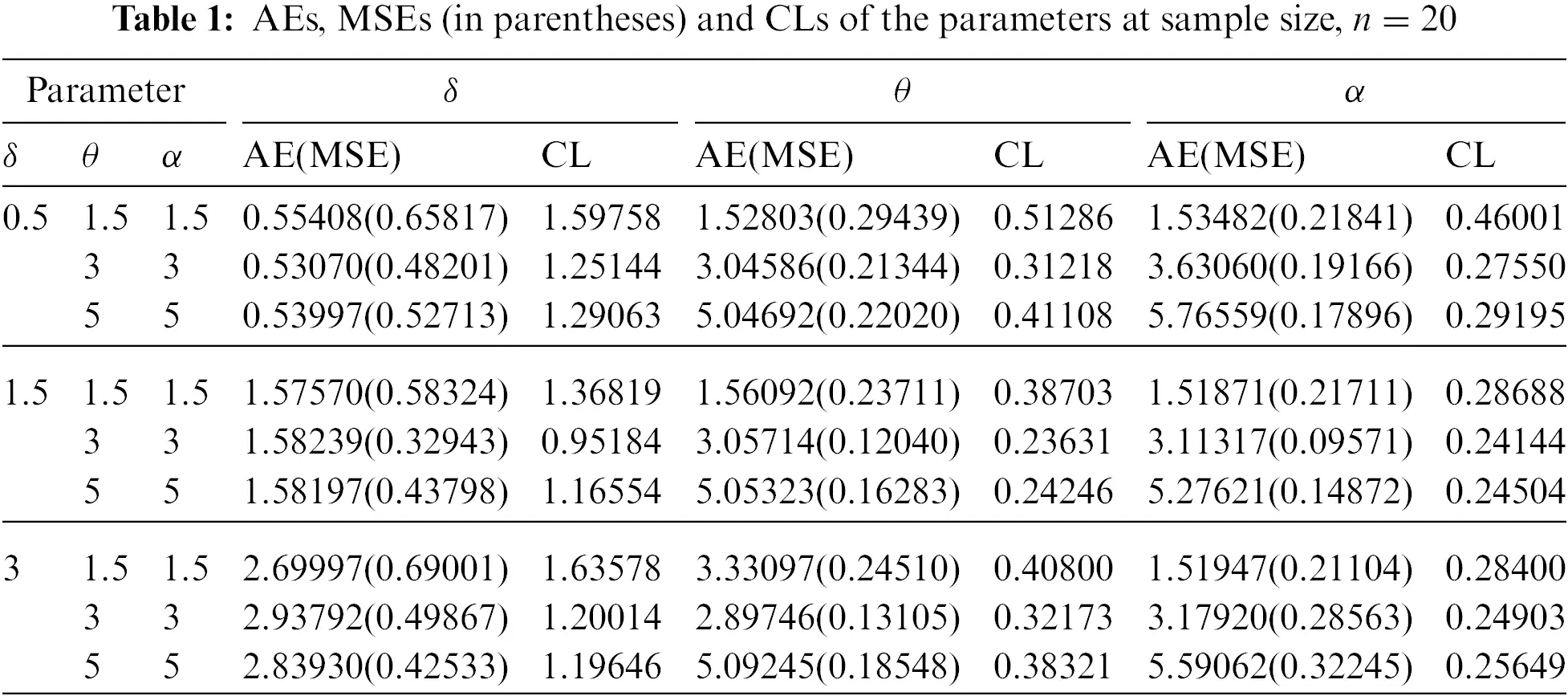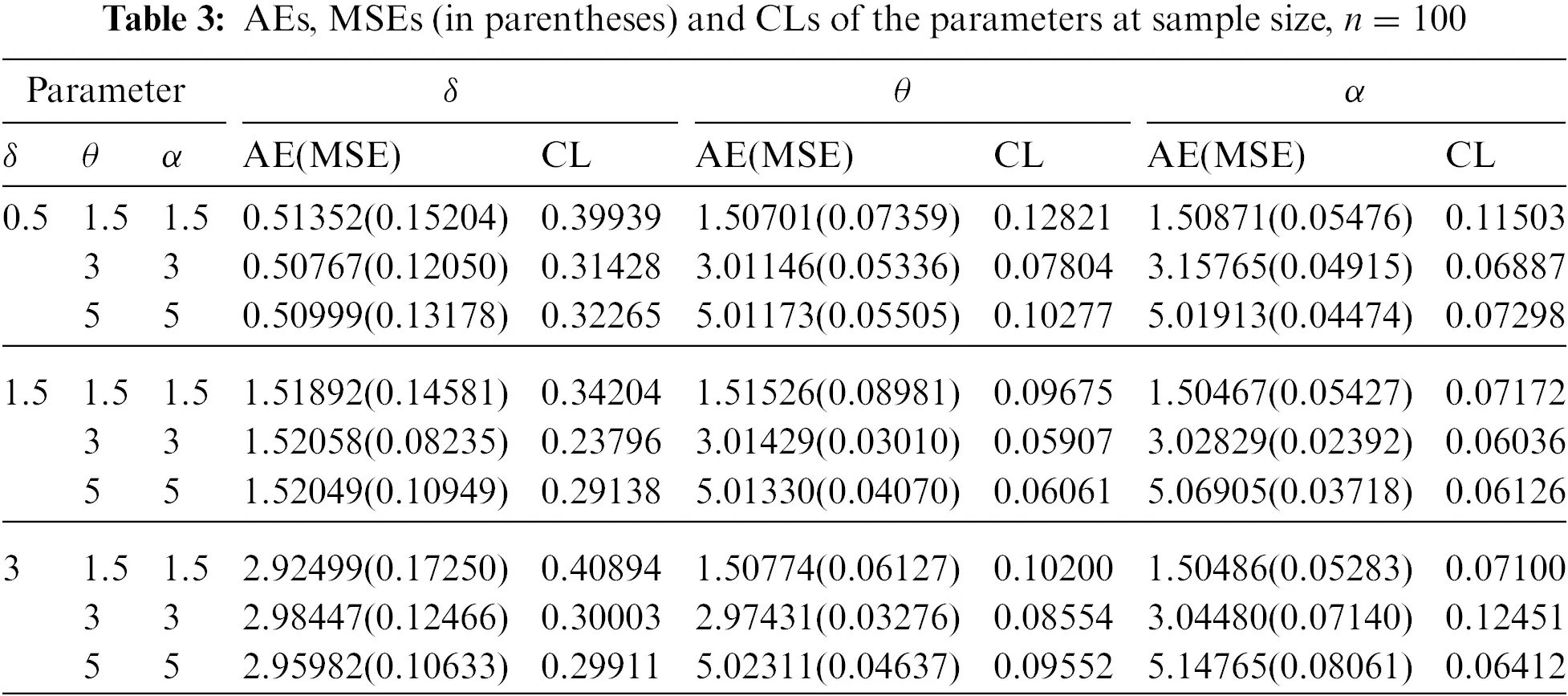6  Applications

In this part, we illustrate the MAPTIW distribution’s flexibility by using three real data sets. The MAPTIW distribution is compared to other models such as, alpha power inverse Lomax (APILo) distribution by ZeinEldin et al. , inverse Lomax (ILo) distribution, alpha power inverse Weibull (APIW) distribution by Basheer , alpha power inverse Lindley (APILi) distribution by Dey et al.  and Weibull (We) distribution. Table 4 displays the PDFs of these distributions.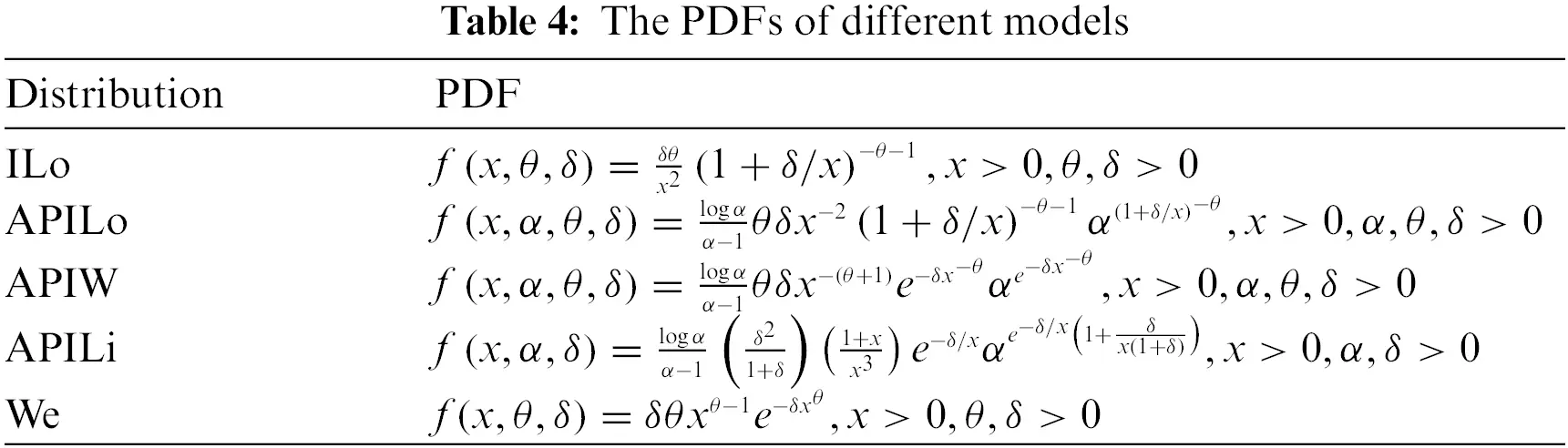The first data (Data 1) describes the mortality rates due to the COVID-19 pandemic in the United Kingdom for 76 days, from 15 April to 30 June 2020. The data is originally investigated by Mubarak et al. . The entire data set is as follows: 0.0587, 0.0863, 0.1165, 0.1247, 0.1277, 0.1303, 0.1652, 0.2079, 0.2395, 0.2751, 0.2845, 0.2992, 0.3188, 0.3317, 0.3446, 0.3553, 0.3622, 0.3926, 0.3926, 0.4110, 0.4633, 0.4690, 0.4954, 0.5139, 0.5696, 0.5837, 0.6197, 0.6365, 0.7096, 0.7193, 0.7444, 0.8590, 1.0438, 1.0602, 1.1305, 1.1468, 1.1533, 1.2260, 1.2707, 1.3423, 1.4149, 1.5709, 1.6017, 1.6083, 1.6324, 1.6998, 1.8164, 1.8392, 1.8721, 1.9844, 2.1360, 2.3987, 2.4153, 2.5225, 2.7087, 2.7946, 3.3609, 3.3715, 3.7840, 3.9042, 4.1969, 4.3451, 4.4627, 4.6477, 5.3664, 5.4500, 5.7522, 6.4241, 7.0657, 7.4456, 8.2307, 9.6315, 10.187, 11.1429, 11.2019, 11.4584.

The second data set (Data 2) describes the remission times (in months) of a random sample of 128 bladder cancer patients studied by Lee et al.  and recently investigated by Al-Zahrani et al. . The complete data set of is: 0.08, 2.09, 3.48, 4.87, 6.94, 8.66, 13.11, 23.63, 0.20, 2.23, 3.52, 4.98, 6.97, 9.02, 13.29, 0.40, 2.26, 3.57, 5.06, 7.09, 9.22, 13.80, 25.74, 0.50, 2.46, 3.64, 5.09, 7.26, 9.47, 14.24, 25.82, 0.51, 2.54, 3.70, 5.17, 7.28, 9.74, 14.76, 26.31, 0.81, 2.62, 3.82, 5.32, 7.32, 10.06, 14.77, 32.15, 2.64, 3.88, 5.32, 7.39, 10.34, 14.83, 34.26, 0.90, 2.69, 4.18, 5.34, 7.59, 10.66, 15.96, 36.66, 1.05, 2.69, 4.23, 5.41, 7.62, 10.75, 16.62, 43.01, 1.19, 2.75, 4.26, 5.41, 7.63, 17.12, 46.12, 1.26, 2.83, 4.33, 5.49, 7.66, 11.25, 17.14, 79.05, 1.35, 2.87, 5.62, 7.87, 11.64, 17.36, 1.40, 3.02, 4.34, 5.71, 7.93, 11.79, 18.10, 1.46, 4.40, 5.85, 8.26, 11.98, 19.13, 1.76, 3.25, 4.50, 6.25, 8.37, 12.02, 2.02, 3.31, 4.51, 6.54, 8.53, 12.03, 20.28, 2.02, 3.36, 6.76, 12.07, 21.73, 2.07, 3.36, 6.93, 8.65, 12.63, 22.69.

The third data set (Data 3) consists of the times of breakdown of a sample of 25 devices at 180°C and given by Pham . The complete data set of is: 112, 260, 298, 327, 379, 487, 593, 658, 701, 720, 734, 736, 775, 915, 974, 1123, 1157, 1227, 1293, 1335, 1472, 1529, 1545, 2029, 4568.

Before studying these data sets, we foremost scheme the corresponding TTT plots. Fig. 3 displays the TTT plots of these data. It indicates that Data 1 has a decreasing failure rate function, while the TTT plots for Data 2 and 3 reveal an upside-down bathtub failure rate function. Therefore, we can infer that the MAPTIW distribution is reasonable to model these data sets. The MLEs and their standard errors (SEs) of the MAPTIW distribution and some other competitive distributions are displayed in Tables 5, 6 and 7 for Data 1, 2 and 3, respectively. In addition, some goodness of fit statistics, namely, Akaike information criterion (AIC), consistent Akaike information criterion (CAIC), Bayesian information criterion (BIC), Hannan-Quinn information criterion (HQIC) statistics, Anderson-Darling (A), Cramér-Von Mises (W) and Kolmogorov-Smirnov (K.S) (with the corresponding p-value) are also presented in Tables 5, 6 and 7 for Data 1, 2 and 3, respectively. It is noted from these Tables that the MAPTIW distribution performed better than all other competitive distributions. As a result, the MAPTIW distribution is determined to be the best model to fit the given two data sets. Figs. 46 display the fitted PDFs, CDFs, RFs and probability-probability (PP) plots of the MAPTIW distribution for Data 1, 2 and 3, respectively. These figures demonstrate that the MAPTIW distribution can furnish a close fit to the given data sets. Generally, we can deduce that the MAPTIW distribution is appropriate for modelling the delivered data sets more preferably than some conventional and some lately suggested models. The ACIs of the unknown parameters of the MAPTIW distribution for Data 1, 2 and 3 are obtained and displayed in Table 8.Figure 3: TTT Plots for Data 1 (left) and Data 2 (middle) and Data 3 (right)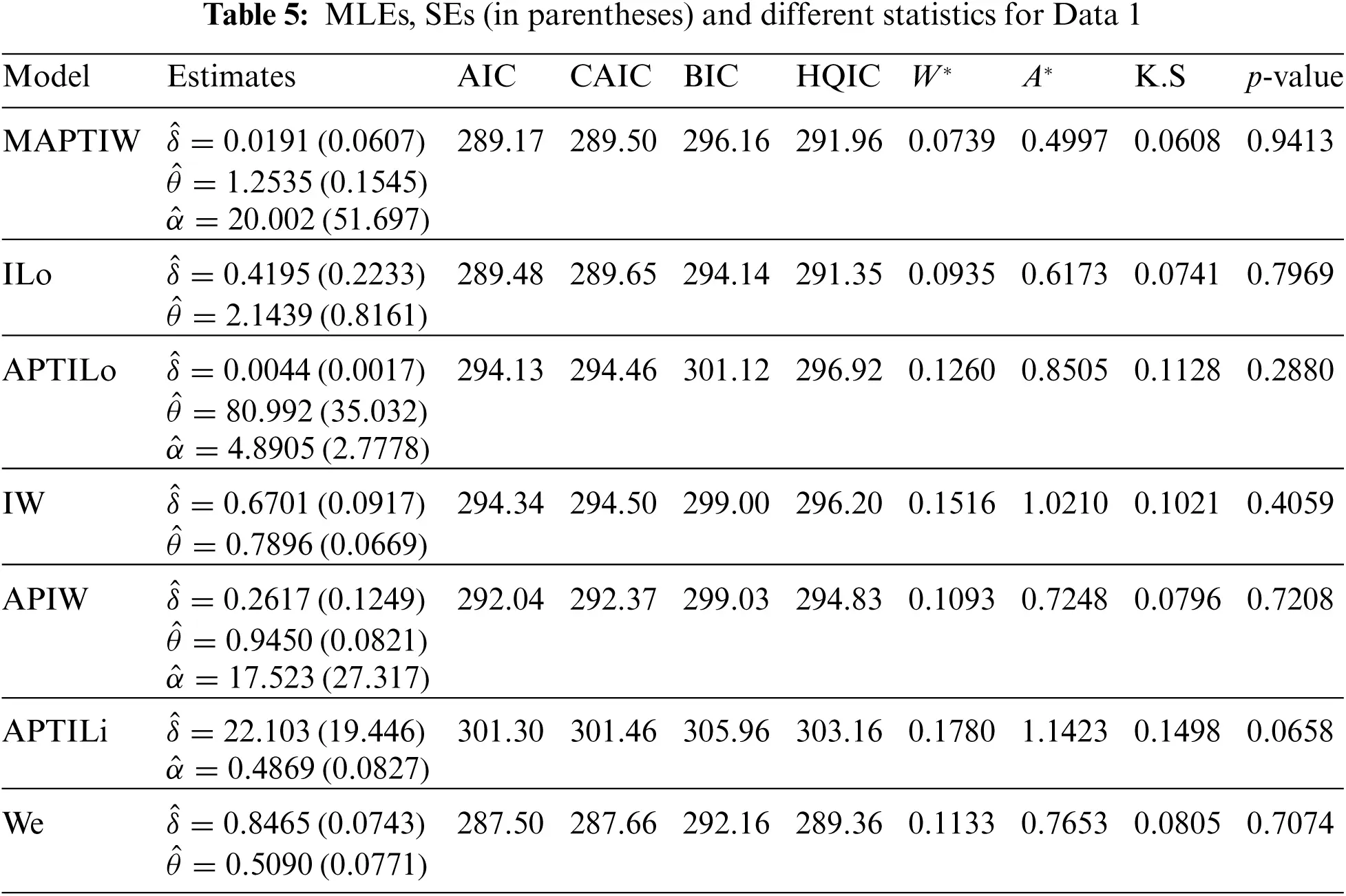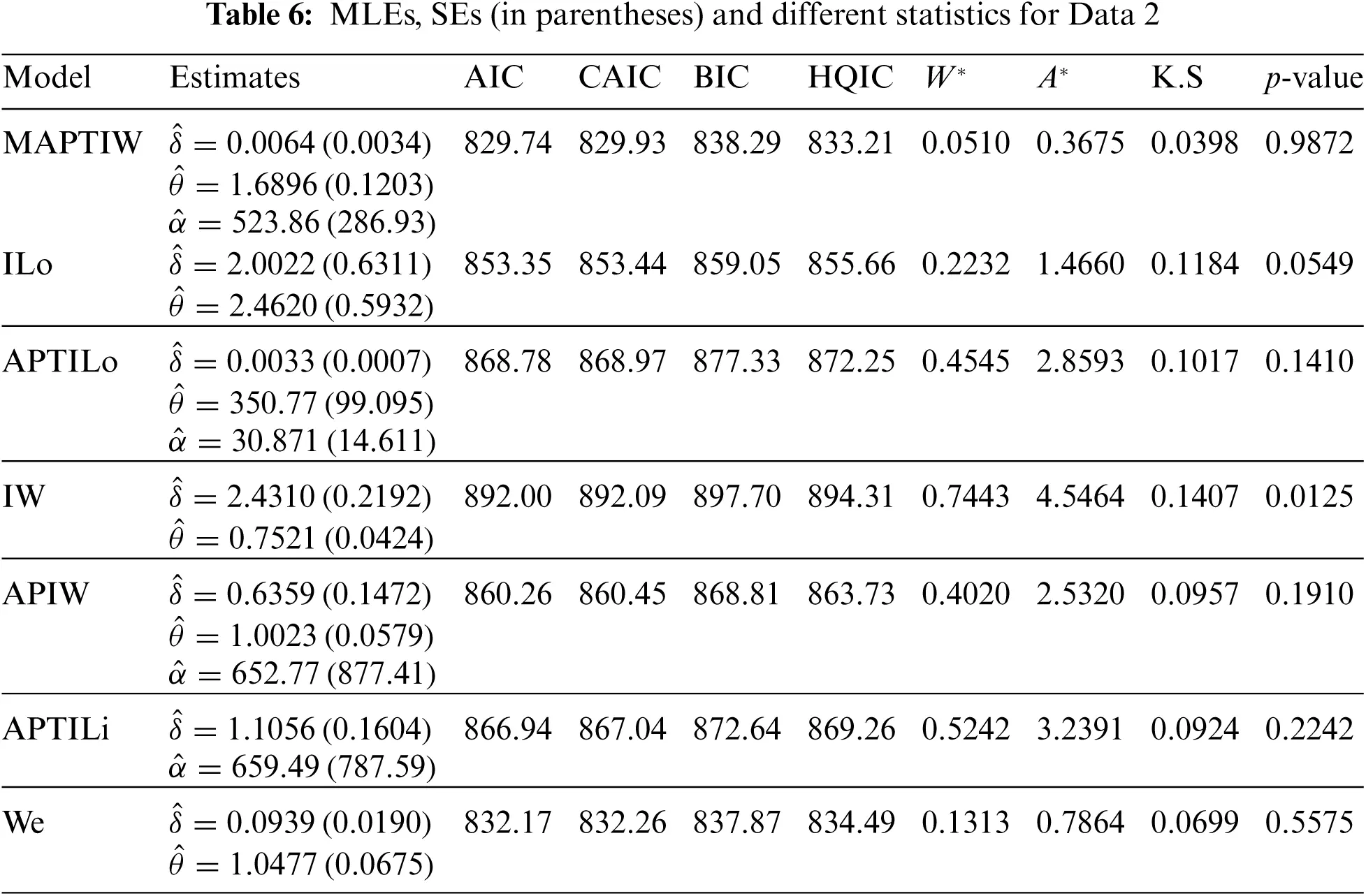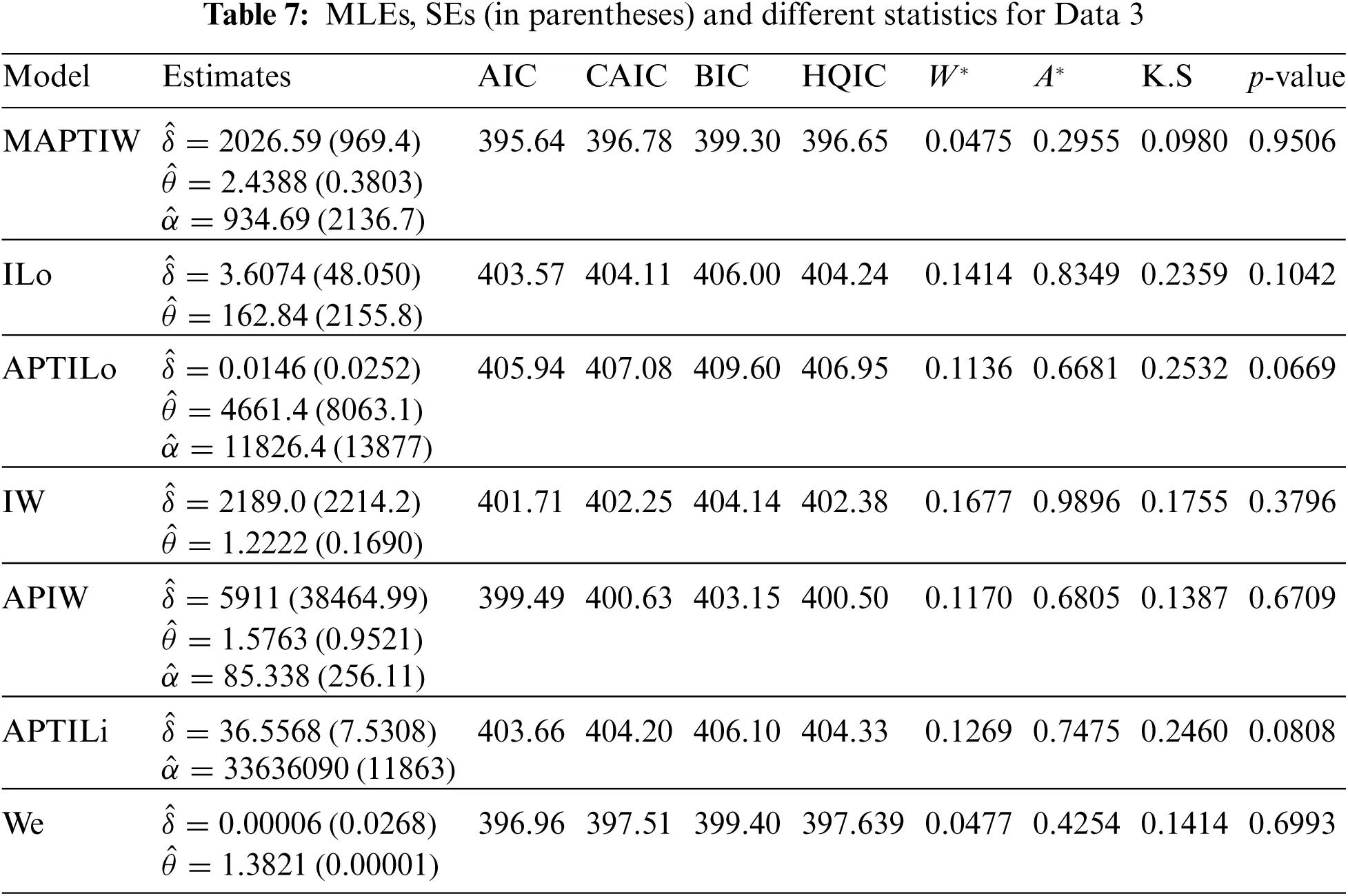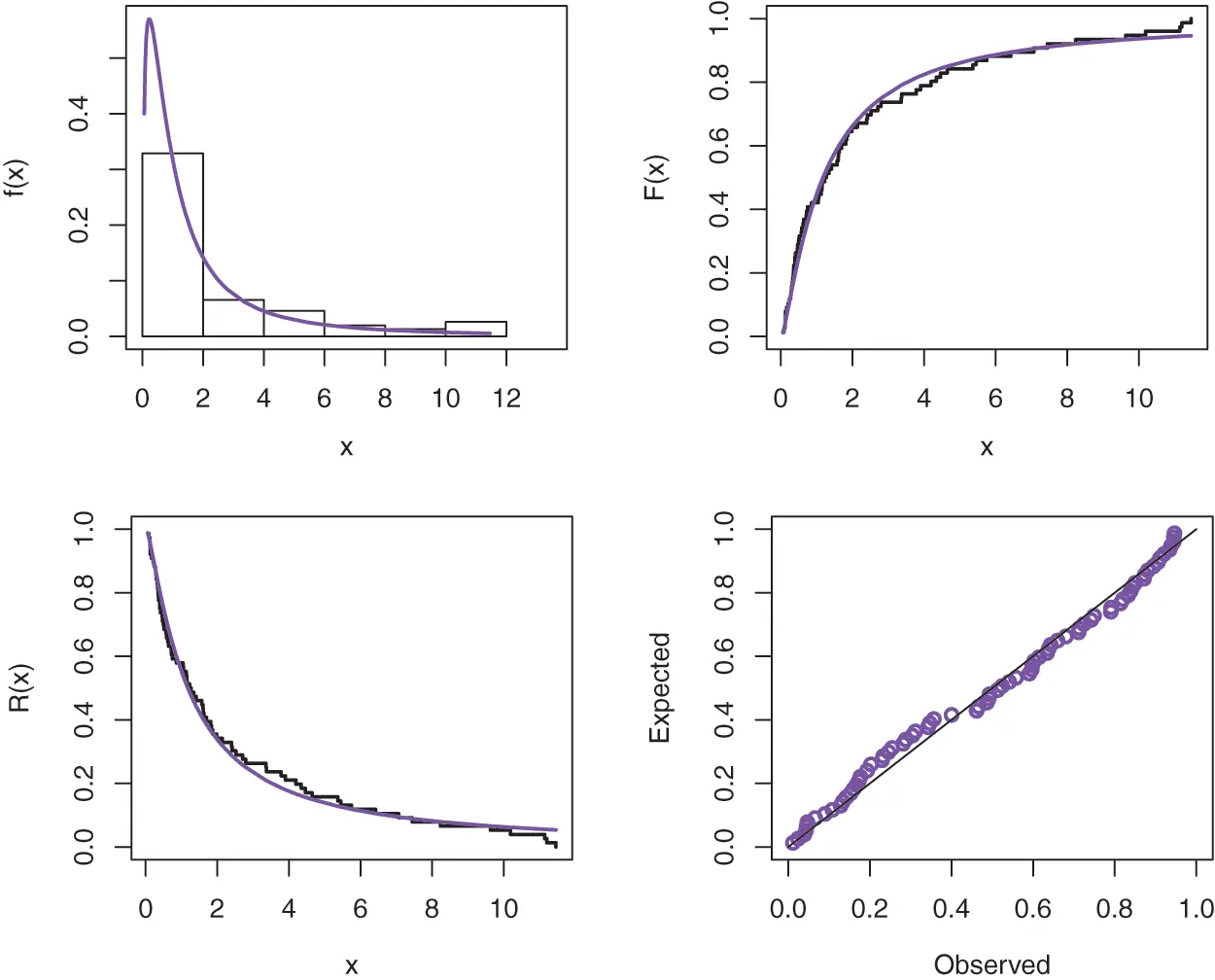Figure 4: Plots of the fitted functions for the MAPTIW distribution and PP plot for Data 1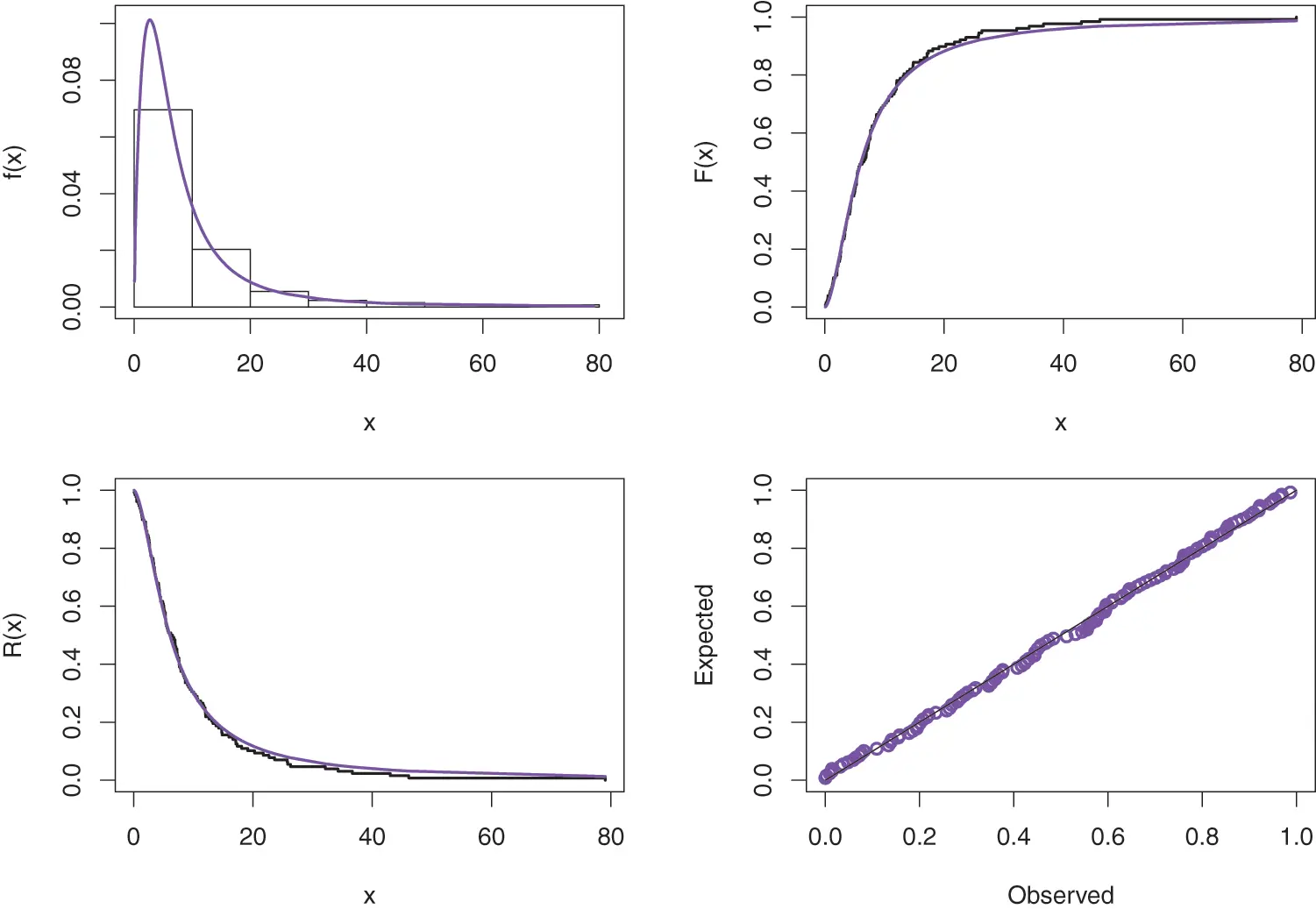Figure 5: Plots of the fitted functions for the MAPTIW distribution and PP plot for Data 2Figure 6: Plots of the fitted functions for the MAPTIW distribution and PP plot for Data 3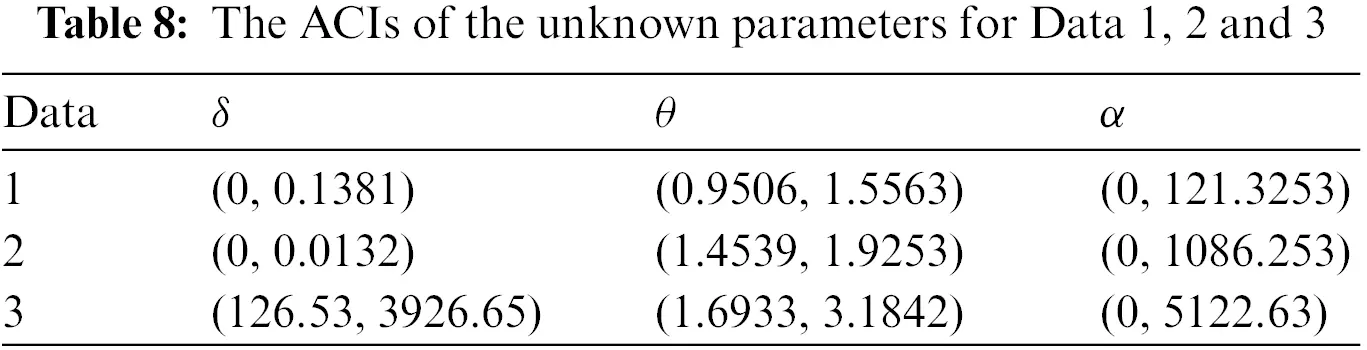7  Conclusion

As a new extension of the inverse Weibull model, we introduced a new three-parameter inverse Weibull distribution. Additionally, numerous theoretical properties of the distribution were explored in order to develop a more flexible model that includes the decreasing and unimodal shape for the hazard rate function. We described the method of maximum likelihood for estimating the parameters of the suggested distribution. A simulation study is also used to investigate the asymptotic behaviour of the maximum likelihood estimators. The model’s efficiency is demonstrated using three real data sets to show its applicability in real life. The proposed distribution is a better distributional model for fitting such data sets than many of its related models, as well as several newly produced distributions, using various information measures.

Acknowledgement: The authors convey their sincere appreciation to the reviewers and the editors for making some valuable suggestions and comments. The authors extend their appreciations to Princess Nourah bint Abdulrahman University Researchers Supporting Project No. (PNURSP2022R50), Princess Nourah bint Abdulrahman University, Riyadh, Saudi Arabia.

Funding Statement: This research was funded by Princess Nourah bint Abdulrahman University Researchers Supporting Project No. (PNURSP2022R50), Princess Nourah bint Abdulrahman University, Riyadh, Saudi Arabia.

Conflicts of Interest: The authors declare that they have no conflicts of interest to report regarding the present study.

## References

1. Lee, C., Famoye, F., & Alzaatreh, A. (2013). Methods for generating families of univariate continuous distributions in the recent decades. WIREs, 5, 219-238. [Google Scholar] [CrossRef]
2. Mudholkar, G. S., & Srivastava, D. K. (1993). Exponentiated weibull family for analyzing bathtub failure-rate data. IEEE Transactions on Reliability, 42, 299-302. [Google Scholar] [CrossRef]
3. Marshall, A. W., & Olkin, I. (1997). A new method for adding a parameter to a family of distributions with application to the exponential and weibull families. Biometrika, 84, 641-652. [Google Scholar] [CrossRef]
4. Mahdavi, A., & Kundu, D. (2017). A new method for generating distributions with an application to exponential distribution. Communications in Statistics–Theory and Methods, 46(13), 6543-6557. [Google Scholar] [CrossRef]
5. Drapella, A. (1993). Complementary weibull distribution: Unknown or just forgotten. Quality and Reliability Engineering International, 9, 383-385. [Google Scholar] [CrossRef]
6. Mudholkar, G. S., & Kollia, G. D. (1994). Generalized weibull family: A structural analysis. Communications in Statistics-Theory and Methods, 23, 1149-1171. [Google Scholar] [CrossRef]
7. Keller, A. Z., & Kamath, A. R. (1982). Reliability analysis of CNC machine tools. Reliability Engineering, 3, 449-473. [Google Scholar] [CrossRef]
8. Khan, M. S. (2010). The beta inverse weibull distribution. International Transactions in Mathematical Sciences and Computer, 3, 113-119. [Google Scholar]
9. de Gusmao, F. R., Ortega, E. M., & Cordeiro, G. M. (2011). The generalized inverse Weibull distribution. Statistical Papers, 52, 591-619. [Google Scholar] [CrossRef]
10. Khan, M. S., & King, R. (2012). Modifieed inverse Weibull distribution. Journal of Statistics Applications & Probability, 1, 115-132. [Google Scholar] [CrossRef]
11. Oluyede, B. O., & Yang, T. (2014). Generalizations of the inverse weibull and related distributions with applications. Electronic Journal of Applied Statistical Analysis, 7, 94-116. [Google Scholar]
12. Aryal, G., & Elbatal, I. (2015). Kumaraswamy modified inverse weibull distribution. Applied Mathematics & Information Sciences, 9, 651-660. [Google Scholar]
13. Okasha, H. M., El-Baz, A. H., Tarabia, A. M. K., & Basheer, A. M. (2017). Extended inverse weibull distribution with reliability application. Journal of the Egyptian Mathematical Society, 25, 343-349. [Google Scholar] [CrossRef]
14. Basheer, A. M. (2019). Alpha power inverseWeibull distribution with reliability application. Journal of Taibah University for Science, 13, 423-432. [Google Scholar] [CrossRef]
15. Dey, S., Nassar, M., Kumar, D., & Alaboud, F. (2019). Logarithm transformed fréchet distribution: Properties and estimation. Austrian Journal of Statistics, 48(1), 70-93. [Google Scholar] [CrossRef]
16. Afify, A. Z., Ahmed, S., & Nassar, M. (2021). A new inverse weibull distribution: Properties, classical and Bayesian estimation with applications. Kuwait Journal of Science, 48(3), [Google Scholar]
17. Johnson, N. L., Kotz, S., Balakrishnan, N. (1995). In: Continuous univariate distributions, 2nd ed., vol. 2. New York, NY, USA: Wiley.
18. Alotaibi, R., Okasha, H., Rezk, H., & Nassar, M. (2021). A new weighted version of alpha power transformation method: Properties and applications to COVID-19 and software reliability data. Physica Scripta, 96, 125-221. [Google Scholar] [CrossRef]
19. Genç, R., & Gradojevic, N. (2017). The tale of two financial crises: An entropic perspective. Entropy, 19(6), 244. [Google Scholar] [CrossRef]
20. Rashidi, S., Akar, S., Bovand, M., & Ellahi, R. (2018). Volume of fluid model to simulate the nanofluid flow and entropy generation in a single slope solar still. Renewable Energy, 115, 400-410. [Google Scholar] [CrossRef]
21. ZeinEldin, R. A., Ahsan ul Haq, M., Hashmi, S., Elsehety, M. (2020). Alpha power transformed inverse lomax distribution with different methods of estimation and applications. Complexity, DOI 10.1155/2020/1860813. [CrossRef]
22. Dey, S., Nassar, M., & Kumar, D. (2019). Alpha power transformed inverse lindley distribution: A distribution with an upside-down bathtub-shaped hazard function. Journal of Computational and Applied Mathematics, 348, 130-145. [Google Scholar] [CrossRef]
23. Mubarak, A. E., Al-Metwally, E. (2021). A New Extension Exponential Distribution with Applications of COVID-19 Data. https://jsst.journals.ekb.eg.
24. Lee, E. T., Wang, J. (2003). Statistical methods for survival data analysis. New Jersey, USA: John Wiley & Sons.
25. Al-Zahrani, B., & Sagor, H. (2015). Statistical analysis of the lomax-logarithmic distribution. Journal of Statistical Computation and Simulation, 85(9), 1883-1901. [Google Scholar] [CrossRef]
26. Shakhatreh, M. K. (2018). A new three-parameter extension of the log-logistic distribution with applications to survival data. Communications in Statistics–Theory and Methods, 47(21), 5205-5226. [Google Scholar] [CrossRef]
27. Klakattawi, H. S. (2022). Survival analysis of cancer patients using a new extended weibull distribution. PLoS One, 17(2), e0264229. [Google Scholar] [CrossRef]
28. Pham, H. (2003). Accelerated life testing. In: Hoang Pham (Ed.), Handbook of reliability engineering, vol. 1. London: Springer.

Alotaibi, R., Okasha, H., Rezk, H., Nassar, M. (2023). A New Three-Parameter Inverse Weibull Distribution with Medical and Engineering Applications. CMES-Computer Modeling in Engineering & Sciences, 135(2), 1255–1274.This work is licensed under a Creative Commons Attribution 4.0 International License , which permits unrestricted use, distribution, and reproduction in any medium, provided the original work is properly cited.

View

Like

## Related articles

• LI KAILONG, DU XIAOLAN, HE YANI,...
• Na Li*, Wei Wang*, Bin Ye*, Song...
• Christopher A. Lemmon, Nathan...
• J. Useche, P. Sollero, E.L. Albuquerque,...
• Girish K., Hema Singh